Get inspired by the success stories of our students in IIT JAM MS, ISI  MStat, CMI MSc DS.  Learn More

# ISI MStat 2018 PSA Problem 12 | Sequence of positive numbersThis is a problem from ISI MStat 2018 PSA Problem 12 based on Sequence of positive numbers

## Sequence of positive numbers - ISI MStat Year 2018 PSA Question 12

Let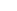,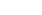be a sequence of positive numbers such that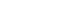for all n, and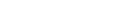Let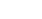be the polynomial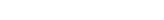and supposehas no real roots for every n . Let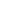and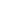be the roots of the polynomial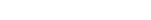What can you say about?

• (A)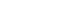andare not real
• (B)andare real.
• (C)andare real.
• (D)andare not real

### Key Concepts

Sequence

Discriminant

ISI MStat 2018 PSA Problem 12

Introduction to Real Analysis by Bertle Sherbert

## Try with Hints

Write the discriminant. Use the properties of the sequence.

Note that as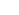"> has no real root so discriminant is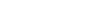"> so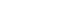"> and">'s are positive and decreasing so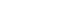"> . So , what can we say about a ?

Therefore we can say that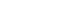hence discriminant of P  ,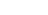must be strictly negative so option D.

## Subscribe to Cheenta at Youtube

This is a problem from ISI MStat 2018 PSA Problem 12 based on Sequence of positive numbers

## Sequence of positive numbers - ISI MStat Year 2018 PSA Question 12

Let,be a sequence of positive numbers such thatfor all n, andLetbe the polynomialand supposehas no real roots for every n . Letandbe the roots of the polynomialWhat can you say about?

• (A)andare not real
• (B)andare real.
• (C)andare real.
• (D)andare not real

### Key Concepts

Sequence

Discriminant

ISI MStat 2018 PSA Problem 12

Introduction to Real Analysis by Bertle Sherbert

## Try with Hints

Write the discriminant. Use the properties of the sequence.

Note that as"> has no real root so discriminant is"> so"> and">'s are positive and decreasing so"> . So , what can we say about a ?

Therefore we can say thathence discriminant of P  ,must be strictly negative so option D.

## Subscribe to Cheenta at Youtube

This site uses Akismet to reduce spam. Learn how your comment data is processed.

### Knowledge Partner